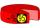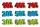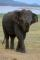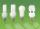Insurance

The owner of the house is insured against natural disasters and pays 0.04% annually of the value of house 77 Eur.

Calculate the value of the house.

Calculate the probability of disaster, if you know that 48% of the insurance is to pay damages.

Result

value of the house:  192500
probability of disaster:  0.019 %

Solution:Leave us a comment of this math problem and its solution (i.e. if it is still somewhat unclear...):Be the first to comment!To solve this verbal math problem are needed these knowledge from mathematics:

Our percentage calculator will help you quickly calculate various typical tasks with percentages. Would you like to compute count of combinations?

Next similar math problems:

1. The percentages in practiceIf every tenth apple on the tree is rotten it can be expressed by percentages: 10% of the apples on the tree is rotten. Tell percent using the following information: a. in June rained 6 days b, increase worker pay 500 euros to 50 euros c, grabbed 21 fro
2. PercentagesExpressed as a percentage:
3. Sales offGoods is worth € 70 and the price of goods fell two weeks in a row by 10%. How many % decreased overall?
4. Conference148 is the total number of employees. The conference was attended by 22 employees. How much is it in percent?
5. Double percentWhat is 80% of 60% of 2800?
6. TVsProduction of television sets increased from 3,500 units to 4,200 units. Calculate the percentage of production increase.
7. Percents - easyHow many percent is 432 out of 434?
8. New refrigeratorNew refrigerator sells for 1024 USD, Monday will be 25% discount. How much USD will save, and what will be the price?
9. 100 %How much is 100%, if 17 % is 1169?
10. ClassIn a class are 32 pupils. Of these are 8 boys. What percentage of girls are in the class?
11. PercentsHow many percents is 900 greater than the number 750?
12. GlovesI have a box with two hundred pieces of gloves in total, split into ten parcels of twenty pieces, and I sell three parcels. What percent of the total amount I sold?
13. Highway repairThe highway repair was planned for 15 days. However, it was reduced by 30%. How many days did the repair of the highway last?
14. Base, percents, valueBase is 344084 which is 100 %. How many percent is 384177?
15. Apples 2James has 13 apples. He has 30 percent more apples than Sam. How many apples has Sam?
16. BulbsIn the box are 6 bulbs with power 75 W, 14 bulbs with power 40 W and 15 with 60 W. Calculate probability that a randomly selected bulb is:
17. ClassIn 7.C clss are 10 girls and 20 boys. Yesterday was missing 20% of girls and 50% boys. What percentage of students missing?